• 定义一个学生类，Student有姓名，学号，选学的课程列表 定义一个课程类课程名称，课程分数 (1)初始化一个学生，选择了三门课程，并添加到学生的属性中 (2)实现统计学生的总分功能 1.正...
package exam2;

import java.util.ArrayList;
import java.util.List;

/**
编写一个Java应用程序，该程序包括3个类：
定义一个学生类，Student有姓名，学号，选学的课程列表
定义一个课程类：课程名称，课程分数
(1)初始化一个学生，选择了三门课程，并添加到学生的属性中
(2)实现统计学生的总分功能

1.正确编写2个类（5分）
2.统计学生的总分功能（5分）
*/
public class Test {
public static void main(String[] args) {

List<Course> courses = new ArrayList<>();

// 初始化3门课程及分数
Course course1 = new Course("java", 80);
Course course2 = new Course("Math", 60);
Course course3 = new Course("English", 90);

// 课程对象添加到集合

// 初始化学生对象
Student student = new Student("tom", "2015101", courses);
// student.setStuid("2015101");
// student.setStuname("tom");
// student.setCourses(courses);

// System.out.println(student);

// (2)实现统计 学生 的总分功能

int sum = sumScore(courses);
System.out.println(student.getStuname() + "学生总分：" + sum);

}

private static int sumScore(List<Course> courses) {
int sum = 0;
// 用课程对象，来获取课程的分数
for (Course course : courses) {
sum += course.getScore();
}
return sum;
}
}
package exam2;

import java.util.List;

public class Student {
// 定义一个学生类，Student有姓名，学号，选学的课程列表
private String stuname;
private String stuid;
private List<Course> courses;

public Student() {
}

public Student(String stuname, String stuid, List<Course> courses) {
this.stuname = stuname;
this.stuid = stuid;
this.courses = courses;
}

public String getStuname() {
return stuname;
}

public void setStuname(String stuname) {
this.stuname = stuname;
}

public String getStuid() {
return stuid;
}

public void setStuid(String stuid) {
this.stuid = stuid;
}

public List<Course> getCourses() {
return courses;
}

public void setCourses(List<Course> courses) {
this.courses = courses;
}

@Override
public String toString() {
return "学生姓名=" + stuname + ",学号=" + stuid + ", 课程=" + courses;
}

}
package exam2;

public class Course {
//定义一个课程类：课程名称，课程分数
private String cname;
private int score;
public Course() {
}
public Course(String cname, int score) {
this.cname = cname;
this.score = score;
}
public String getCname() {
return cname;
}
public void setCname(String cname) {
this.cname = cname;
}
public int getScore() {
return score;
}
public void setScore(int score) {
this.score = score;
}
@Override
public String toString() {
return "[课程名称=" + cname + ", 课程分数=" + score + "]";
}

}


/*
运行：
tom学生总分：230
*/


展开全文• 设计一个Windows应用程序，在该程序中定义一个学生类和班级，以处理每个学生的学号、姓名、语文、数学英语3门课程的期末考试成绩，要求： （1）能根据姓名查询指定学生的总成绩。 （2）能统计全班学生的平均...
设计一个Windows应用程序，在该程序中定义一个学生类和班级类，以处理每个学生的学号、姓名、语文、数学和英语3门课程的期末考试成绩，要求：
（1）能根据姓名查询指定学生的总成绩。
（2）能统计全班学生的平均成绩。
（3）能统计单科成绩最高分。
（4）能统计全班前3名的名单。
（5）能统计指定课程不及格的学生名单。
（6）能统计指定课程在不同分数段的学生人数百分百。
设计提示：
（1）定义一个Student学生类，包含字段（学号、姓名、语文成绩、数学成绩、英语成绩）和属性（总成绩）等。
（3）设计用户操作界面，首先让用户能输入一个学生的信息，当单击“添加”按钮时把这些信息添加到班级对象的学生数组中。单击“完成”按钮调用班级类的方法来显示各种统计结果。当用户输入了学生姓名并且单击“查询”按钮时显示该学生的总成绩。
首先设计如下界面：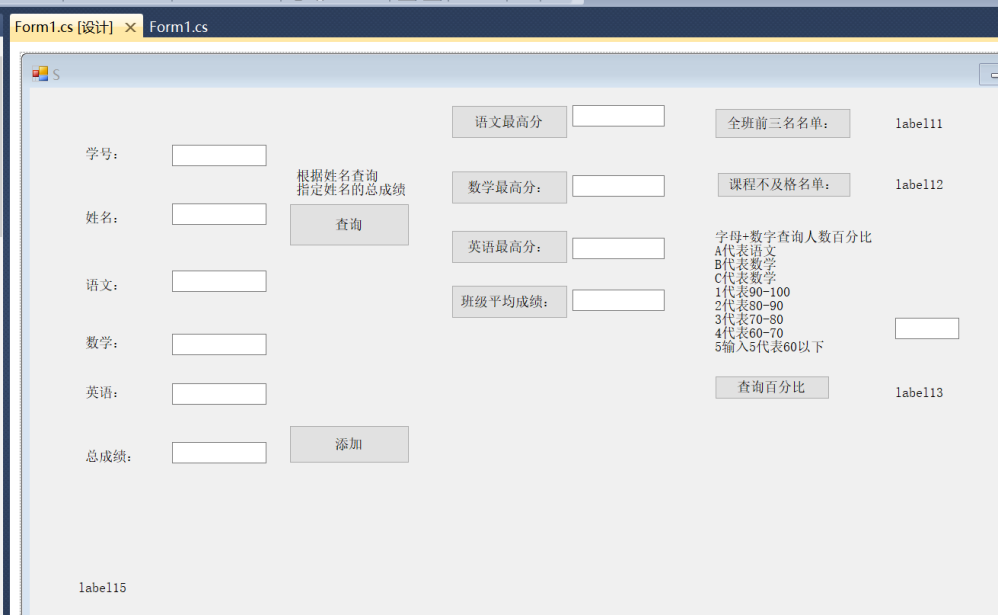编写如下代码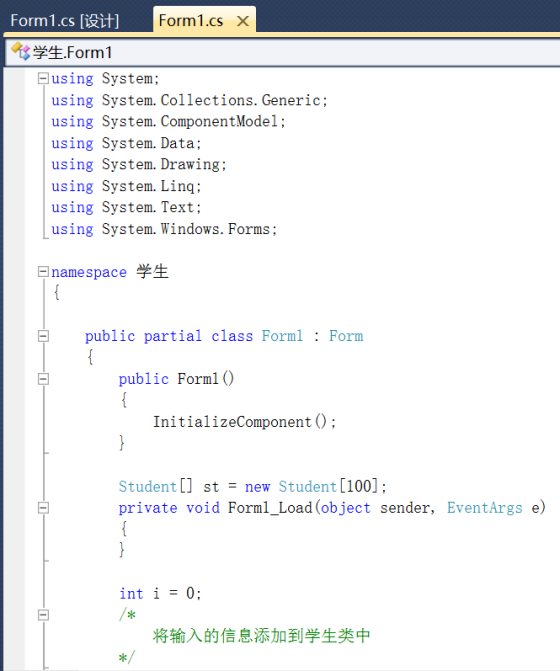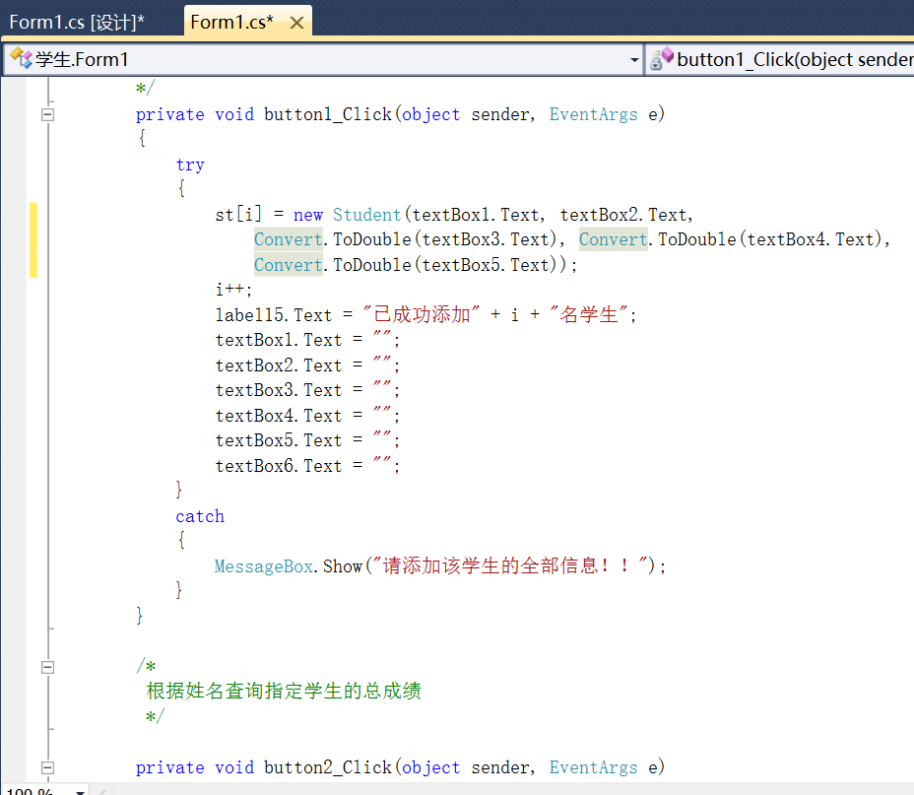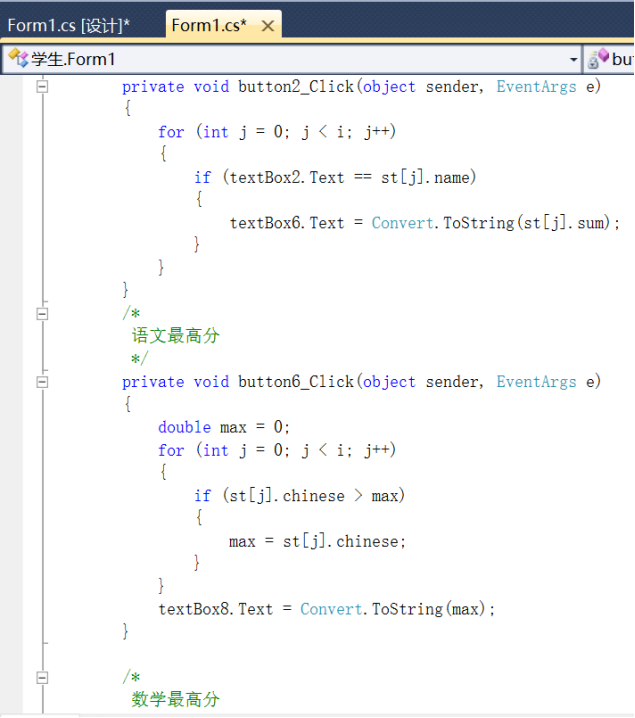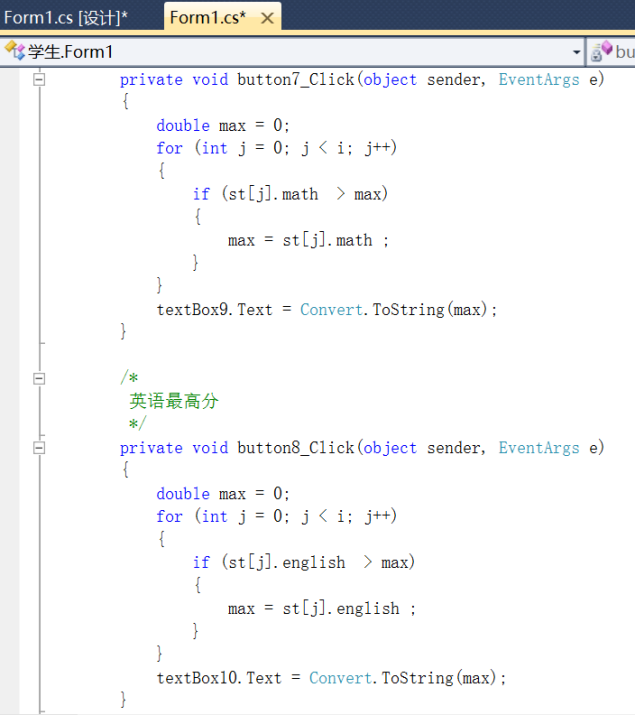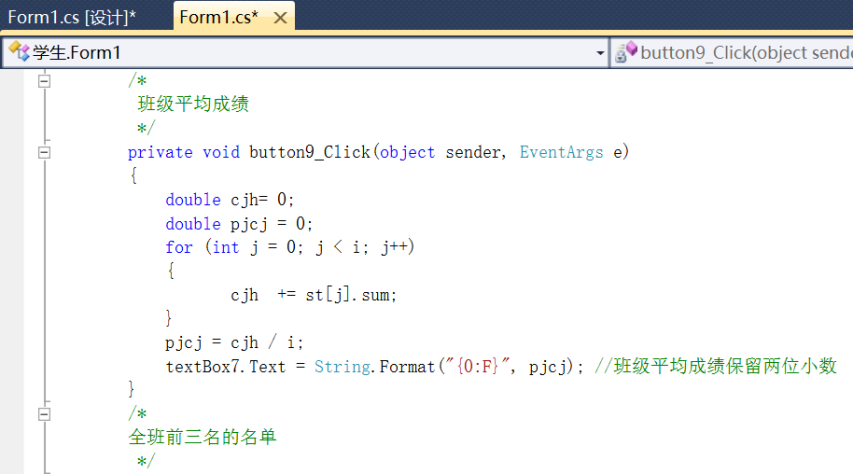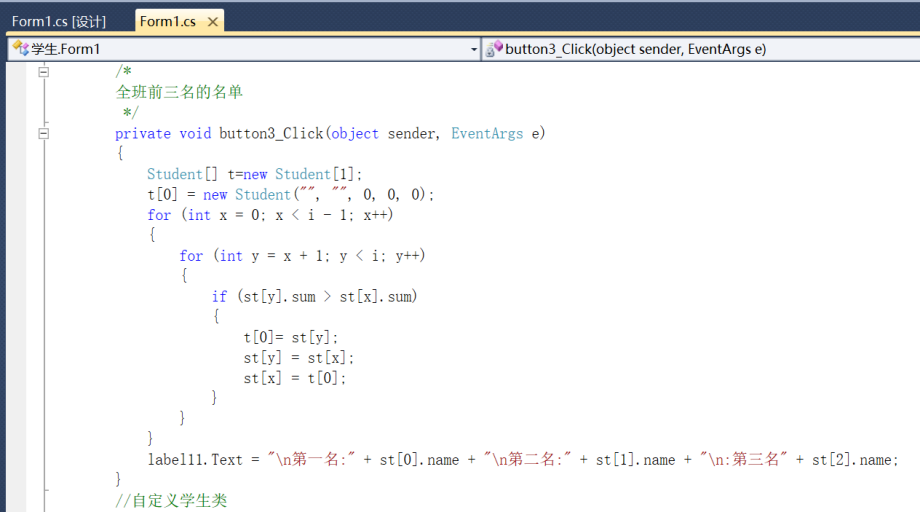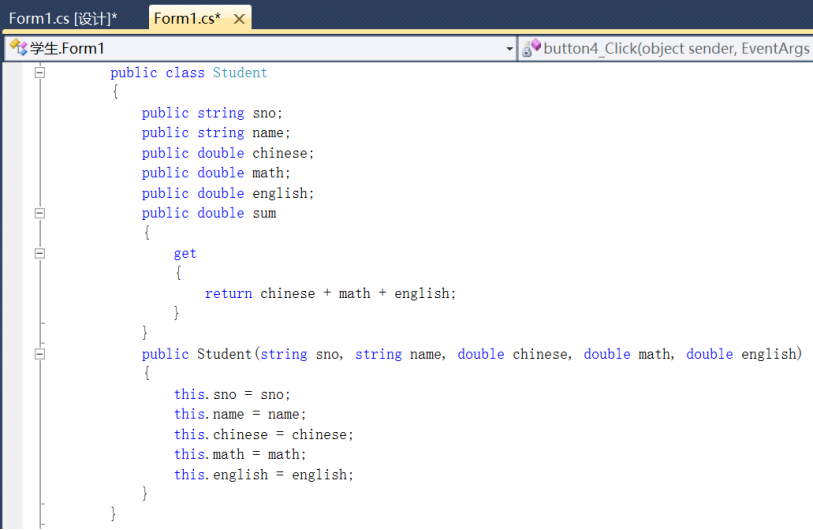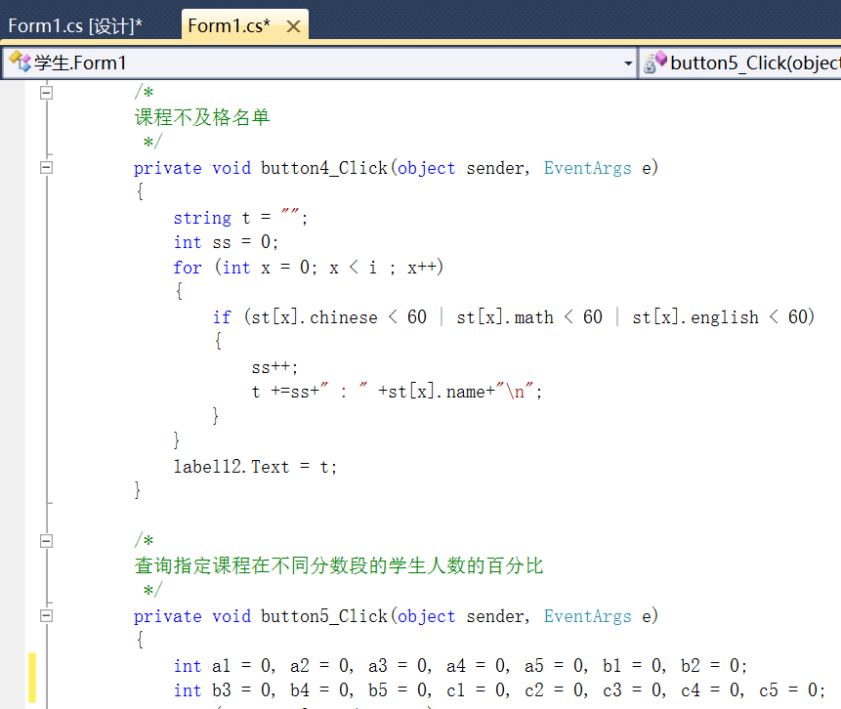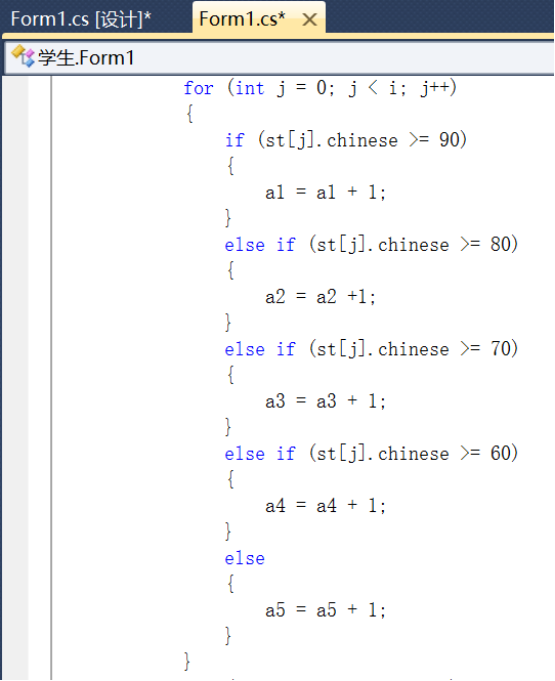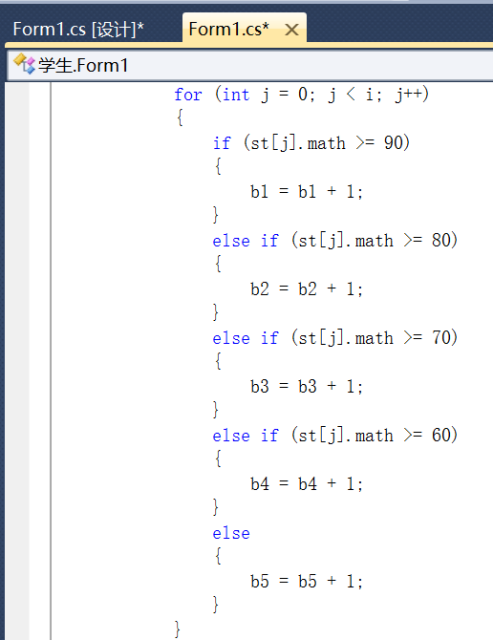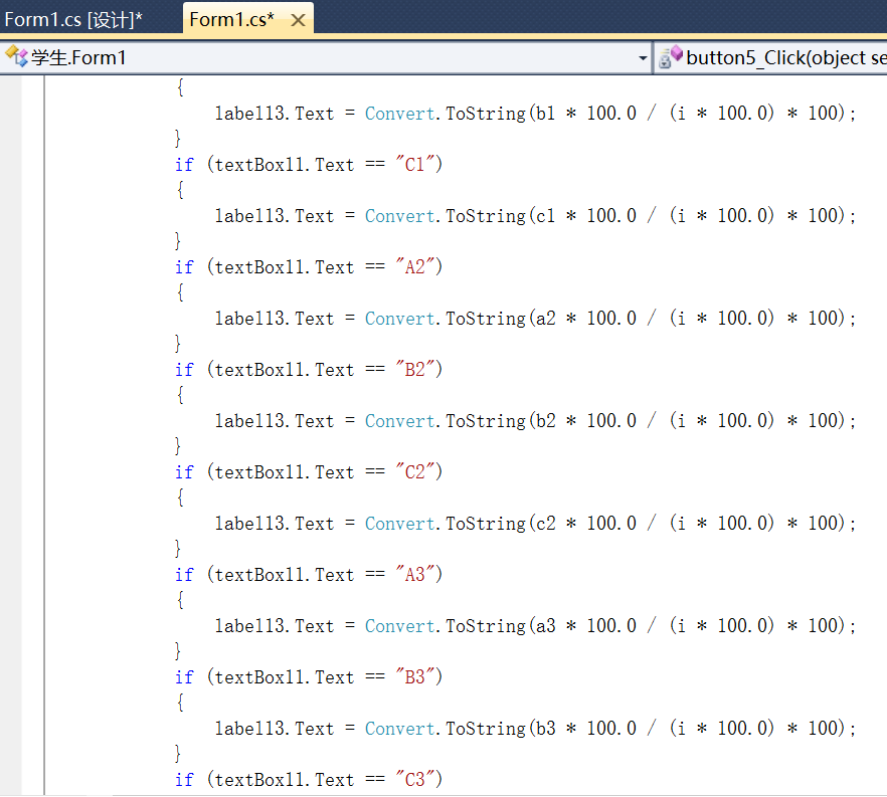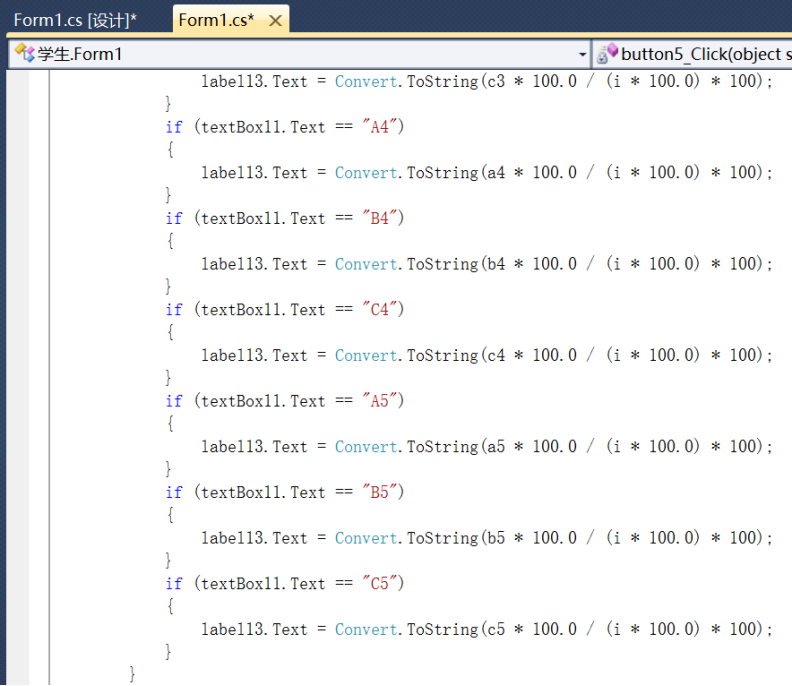展开全文• 设计一个Windows应用程序，在该程序中定义一个学生类和班级，以处理每个学生的学号、姓名、语文、数学英语3门课程的期末考试成绩，要求： （1）能根据姓名查询指定学生的总成绩。 （2）能统计全班学生的平均...
设计一个Windows应用程序，在该程序中定义一个学生类和班级类，以处理每个学生的学号、姓名、语文、数学和英语3门课程的期末考试成绩，要求：
（1）能根据姓名查询指定学生的总成绩。
（2）能统计全班学生的平均成绩。
（3）能统计单科成绩最高分。
（4）能统计全班前3名的名单。
（5）能统计指定课程不及格的学生名单。
（6）能统计指定课程在不同分数段的学生人数百分百。
设计提示：
（1）定义一个Student学生类，包含字段（学号、姓名、语文成绩、数学成绩、英语成绩）和属性（总成绩）等。
（3）设计用户操作界面，首先让用户能输入一个学生的信息，当单击“添加”按钮时把这些信息添加到班级对象的学生数组中。单击“完成”按钮调用班级类的方法来显示各种统计结果。当用户输入了学生姓名并且单击“查询”按钮时显示该学生的总成绩。

设计界面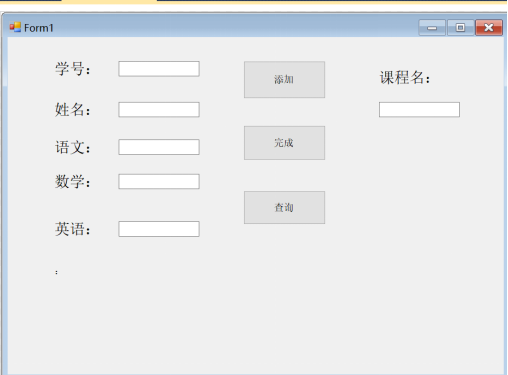编写代码

using System;
using System.Collections.Generic;
using System.ComponentModel;
using System.Data;
using System.Drawing;
using System.Linq;
using System.Text;
using System.Windows.Forms;

namespace c
{
public partial class Form1 : Form
{
public Form1()
{
InitializeComponent();
}

private void Form1_Load(object sender, EventArgs e)
{
label7.Text = "";
}
Student []st1=new Student;
int i = 0;

//添加学生信息
private void button1_Click(object sender, EventArgs e)
{
try
{
st1[i] = new Student(textBox1.Text, textBox2.Text, Convert.ToInt32(textBox3.Text), Convert.ToInt32(textBox4.Text), Convert.ToInt32(textBox5.Text));
i++;
label7.Text = "成功添加" + i + "个学生的信息";
textBox1.Text = "";
textBox2.Text = "";
textBox3.Text = "";
textBox4.Text = "";
textBox5.Text = "";
textBox6.Text = "";
}
catch
{
MessageBox.Show("输入完善的学生信息进行添加！！！");
}
}

//点击完成按钮显示各种查询结果
private void button2_Click(object sender, EventArgs e)
{
label7.Text = "";
if (textBox6.Text.Trim() != "")
{
//（2）可以统计全班学生的平均成绩
label7.Text += "\n\n全班同学的平均成绩为:   " + Convert.ToString(gr.getAverage());

//（3）可以统计单科成绩的最高分
label7.Text += "\n\n语文成绩最高分为：" + Convert.ToString(gr.getChineseMax());
label7.Text += "\n\n数学成绩最高分为：" + Convert.ToString(gr.getMathMax());
label7.Text += "\n\n英语成绩最高分为：" + Convert.ToString(gr.getEnglishMax());

//（4）可以统计全班前3名的名单
label7.Text += "\n\n全班前三名的名单为：" + gr.getNames();

//（5）可以指定课程不及格的学生名单
label7.Text += "\n\n指定科目“ " + textBox6.Text + " ”   不及格的名单：" + gr.getStudentMenu(textBox6.Text);

//（6）可以统计指定课程在不同分数段的学生人数百分比
label7.Text += "\n\n指定科目“ " + textBox6.Text + " ”   不同分数段的百分比如下：" + gr.getStudentBFB(textBox6.Text);
textBox6.Text = "";
}
else
{
MessageBox.Show("请输入您要查询的课程名称：");
}

}

//（1）可以根据姓名查询指定学生的总成绩
private void button3_Click(object sender, EventArgs e)
{
label7 .Text ="";
if (textBox2.Text.Trim() != "")
{
double result = gr.getSum(textBox2.Text);
if (result == -1)
{
MessageBox.Show("该学生不存在！！！");
}
else
{
label7.Text = "学生：" + textBox2.Text + "   的总成绩为：" + Convert.ToString(result);
textBox2.Text = "";
}
}
else
{
MessageBox.Show("请输入您要查询的学生姓名");
}
}

}
public class Student
{
public string sno;
public string name;
public double chinese;
public double math;
public double english;
public Student(string sno,string name,double chinese,double math,double english)
{
this.sno = sno;
this.name = name;
this.chinese = chinese;
this.math = math;
this.english = english;
}
public double Sum
{
get {
return chinese +math +english ;
}
}
}

{
Student [] stu=new Student;  //存放班级中的每个同学的信息
double [] sum2=new double;  //存放每个同学的总成绩
int i = 0; //学生人数

{
stu[i] = s;  //添加每个学生到班级中
sum2[i] = stu[i].Sum;  //保存每个学生的总成绩
i++;  //学生人数加1
}
//查询指定学生的总成绩（1）
int x = 0;
int k = 0;
bool flag = false;
public double getSum(string s)
{
for (x = 0; x < i;x++ )
{
if(stu[x].name==s)
{
k = x;
flag = true;
}
}
if (flag == true)
{
return sum2[k];  //如果该学生在班级中，则返回该学生的总成绩，否则，返回-1
}
else
{
return -1;
}
}

//统计全班同学的平均成绩(2)
double  avg = 0;
public double getAverage()
{
for (int aa = 0; aa < i;aa++ )
{
avg += sum2[aa];
}
return avg/i;
}

//统计语文成绩最高分（3—1）
double  maxChinese = 0;
public double getChineseMax()
{
for (int z = 0; z < i;z++ )
{
if(stu[z].chinese>maxChinese)
{
maxChinese = stu[z].chinese;
}
}
return maxChinese;
}

//统计数学成绩最高分（3—2）
double maxMath = 0;
public double getMathMax()
{
for (int z = 0; z < i; z++)
{
if (stu[z].math > maxMath)
{
maxMath = stu[z].math;
}
}
return maxMath;
}

//统计英语成绩最高分（3—3）
double maxEnglish = 0;
public double getEnglishMax()
{
for (int z = 0; z < i; z++)
{
if (stu[z].english > maxEnglish)
{
maxEnglish = stu[z].english;
}
}
return maxEnglish;
}

//可以统计全班前3名的名单（4）——根据总成绩数组sum2[]将所有学生即stu数组重新排序
public string getNames()
{
Student []t=new Student;  //中间变量（通过Student类型的中间变量，根据每个学生的总成绩重新排列学生类的全部信息）
t=new Student("","",0,0,0);
double t2 = 0;
for(int xx=0;xx<i-1;xx++)
{
for (int yy = xx+1; yy < i;yy++ )
{
if (sum2 [yy]>sum2 [xx])
{
t2=sum2[yy];
sum2[yy] = sum2[xx];
sum2[xx] = t2;
t = stu[yy];
stu[yy] = stu[xx];
stu[xx] = t;
}
}
}
return " " + stu.name + " " + stu.name + " " + stu.name;
}

//可以指定课程不及格的学生名单（5）
string md = "";
{
if(s=="语文")
{
for (int x = 0; x < i;x++ )
{
if(stu [x].chinese <60)
{
md += " "+stu[x].name;
}
}
return " "+md;
}
else if(s=="数学")
{
for (int x = 0; x < i; x++)
{
if (stu[x].math < 60)
{
md += " " + stu[x].name;
}
}
return " " + md;
}
else if(s=="英语")
{
for (int x = 0; x < i; x++)
{
if (stu[x].english < 60)
{
md += " " + stu[x].name;
}
}
return " " + md;
}
else
{
return "不存在（您输入的课程名称不正确）";
}
}

//可以统计指定课程在不同分数段的学生人数百分比（6）
public string getStudentBFB(string s)
{
if(s=="语文")
{
double yw1 = 0; double yw2 = 0; double yw3 = 0; double yw4 = 0; double yw5 = 0;
for (int z = 0; z < i;z++ )
{
if(stu[z].chinese <=100&&stu[z].chinese>=90)
{
yw1++;
}
else if(stu[z].chinese <90&&stu[z].chinese>=80)
{
yw2++;
}
else if (stu[z].chinese < 80 && stu[z].chinese >= 70)
{
yw3++;
}
else if (stu[z].chinese < 70 && stu[z].chinese >= 60)
{
yw4++;
}
else
{
yw5++;
}
}
return "\n90-100:" + (yw1 / i) * 100.0 + "%\n80-90:"  + (yw2 / i) * 100.0 + "%\n70-80:"+ (yw3 / i) * 100.0
+ "%\n60-70:" + (yw4 / i) * 100.0 + "%\n60以下:"+ (yw5 / i) * 100.0 + "%";
}
else if(s=="数学")
{
double yw1 = 0; double yw2 = 0; double yw3 = 0; double yw4 = 0; double yw5 = 0;
for (int z = 0; z < i; z++)
{
if (stu[z].chinese <= 100 && stu[z].chinese >= 90)
{
yw1++;
}
else if (stu[z].chinese < 90 && stu[z].chinese >= 80)
{
yw2++;
}
else if (stu[z].chinese < 80 && stu[z].chinese >= 70)
{
yw3++;
}
else if (stu[z].chinese < 70 && stu[z].chinese >= 60)
{
yw4++;
}
else
{
yw5++;
}
}
return "\n90-100:" + (yw1 / i) * 100.0 + "%\n80-90:" + (yw2 / i) * 100.0 + "%\n70-80:" +
(yw3 / i) * 100.0 + "%\n60-70:" + (yw4 / i) * 100.0 + "%\n60以下:" + (yw5 / i) * 100.0 + "%";
}
else if (s == "英语")
{
double yw1 = 0; double yw2 = 0; double yw3 = 0; double yw4 = 0; double yw5 = 0;
for (int z = 0; z < i; z++)
{
if (stu[z].chinese <= 100 && stu[z].chinese >= 90)
{
yw1++;
}
else if (stu[z].chinese < 90 && stu[z].chinese >= 80)
{
yw2++;
}
else if (stu[z].chinese < 80 && stu[z].chinese >= 70)
{
yw3++;
}
else if (stu[z].chinese < 70 && stu[z].chinese >= 60)
{
yw4++;
}
else
{
yw5++;
}
}
return "\n90-100:" + (yw1 / i) * 100.0 + "%\n80-90:" + (yw2 / i) * 100.0 + "%\n70-80:" +
(yw3 / i) * 100.0 + "%\n60-70:" + (yw4 / i) * 100.0 + "%\n60以下:" + (yw5 / i) * 100.0 + "%";
}
else
{
return "不存在（您输入的课程名称不正确）";
}
}

}
}

运行结果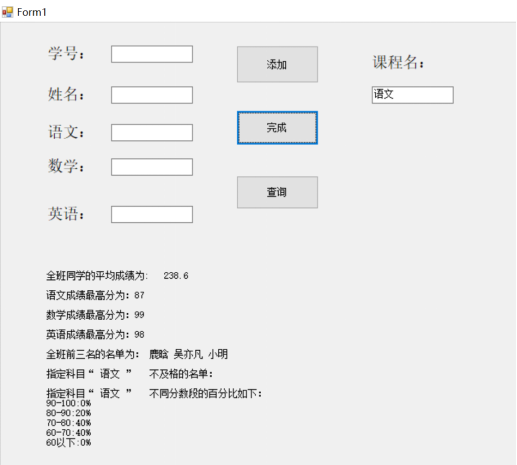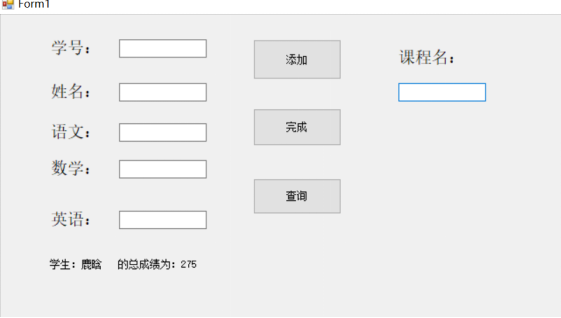展开全文C#
• 编写一个表达学生和课程关系的程序， 定义两个StudentCourse.在Student中有一个Course的数组，表示学生所选的课程;在Course里有一个Student数组，表示选了该课程学生。程序首先要求用户输入课程的数量，...
编写一个表达学生和课程关系的程序，
定义两个类Student和Course.在Student类中有一个Course的数组，表示学生所选的课程;在Course类里有一个Student数组，表示选了该课程的学生。程序首先要求用户输入课程的数量，然后依此输入所有课程的名称;接着要求用户输入学生的数量，然后依次输入学生的信息。先输入学生的姓名，再输入该生选课的数量，接着输入该生选择的每一门课的名称。在输入的过程中，将信息填入相应的Student和Course对象中。最后打印出每个学生所选的所有的课程列表和每个课程的所有选课的学生的列表。
有同学问我，这道题目，随手写出来的代码，没优化。
package chen;

import java.util.ArrayList;
import java.util.Arrays;
import java.util.Scanner;

class Stu {
private String name;//姓名
private int numberCourse; //选课数量
private String[] myCourse = new String;  // 每个人最多10个课程

public Stu(String name, int number, String[] course) {
super();
this.name = name;
this.numberCourse = number;
this.myCourse = course;
}

public String getName() {
return name;
}

public void setName(String name) {
this.name = name;
}

public void printCourse() {
System.out.println(Arrays.toString(this.myCourse));
//        for(int a:array)
//            System.out.println(a);
}
}

class Course {
private String name;//姓名
private int numberStu = 0; //学生数量
private String[] myStu = new String;  // 每个课最多100个学生

public Course(String name) {
super();
this.name = name;
}

myStu[numberStu] = stuName;
numberStu++;
}

public String getName() {
return name;
}

public void printStu() {
System.out.println(this.name + "::");
if (numberStu > 0) {
for (int i = 0; i < numberStu; i++) {
System.out.println(myStu[i]);
}
} else {
System.out.println("此课程无人选择!");
}
System.out.println();

//        System.out.println(Arrays.toString(this.myStu));
//        for(int a:array)
//            System.out.println(a);
}
}

//class init {
//    public init (){
//        System.out.println("初始化...请输入");
//    }
//}

public class ChenBaby {
static ArrayList<String> stu_arr_all = new ArrayList<>();
static ArrayList<String> course_arr_all = new ArrayList<>();
static Stu[] stuArr = new Stu;
static Course[] courseArr = new Course;
static int numberCourse;
static int numberStu;

public static void main(String[] args) {
// 课程输入 初始化 courseArr数组
System.out.println("初始化...请输入所有课程的数量");
Scanner scan = new Scanner(System.in);
numberCourse = scan.nextInt();
System.out.println(numberCourse);
Scanner sc = new Scanner(System.in);
System.out.println("初始化...请输入" + numberCourse + "个名称：");
for (int i = 0; i < numberCourse; i++) {
}
for (int i = 0; i < numberCourse; i++) {
courseArr[i] = new Course(course_arr_all.get(i));
}
//        String[] str = sc.nextLine().split(" "); //字符串以空格分割
System.out.println(course_arr_all);

// 学生输入 初始化 stuArr数组
System.out.println("初始化...请输入所有学生的数量");
numberStu = scan.nextInt();
System.out.println(numberStu);
for (int i = 0; i < numberStu; i++) {
System.out.println("初始化...请输入第" + (i + 1) + "个名字，还剩" + (numberStu - i - 1) + "个学生待输入");
String name = sc.nextLine();
System.out.println("初始化...请输入" + name + "学生选课的数量");
String number = sc.nextLine();
System.out.println("初始化...请输入该学生所有选课的名称");
ArrayList<String> courses = new ArrayList<>();
for (int j = 0; j < Integer.parseInt(number); j++) {
}
// 遍历此学生的输入课程 添加学生到课程中
for (String coursesName : courses) {
for (int z = 0; z < numberCourse; z++) {
//                    System.out.println(courseArr[z].getName());
if (coursesName.equals(courseArr[z].getName())) {
}

}
}
Stu stu = new Stu(name, Integer.parseInt(number), courses.toArray(new String));//这个toArray()方法，而不传递任何参数返回Object[]..因此，必须将数组作为参数传递，该数组将被列表中的数据填充并返回。
stuArr[i] = stu;

}
sc.close();
scan.close();
System.out.println("=============初始化成功===============");
System.out.println("=============最爱陈baby===============");

// 输出
for (int i = 0; i < numberStu; i++) {
System.out.println();
System.out.println(stuArr[i].getName() + "的选课是：");
stuArr[i].printCourse();
}
System.out.println();
System.out.println("(::后面是选课学生名::前面是课名)");
for (int i = 0; i < numberCourse; i++) {
courseArr[i].printStu();
}

}
}


展开全文• 设计一个Windows应用程序，在该程序中定义一个学生类和班级，以处理学生的学号，姓名，语文，数学英语3门课程的期末考试成绩。实现如下要求的功能： 根据姓名查询该学生的总成绩； 统计全班学生总分的平均成绩...
• 定义一个表示学生信息的Student，要求如下： （1）Student的成员变量：  nO 表示学号；name表示姓名；sex表示性别；age表示年龄；java：表示Java课程成绩。 （2）Student带参数的构造方法：  在构造...java Student
• 定义一个表示学生信息的Student，要求如下： （1）Student的成员变量： sNO　表示学号；sName表示姓名；sSex表示性别；sAge表示年龄；sJava：表示Java课程成绩。 （2）Student带参数的构造方法： 在构造方法中...java
• 定义一个表示学生信息的Student，要求如下：（有关的编程） 　（1）Student的成员变量： sNO　表示学号；sName表示姓名；sSex表示性别；sAge表示年龄；sJava：表示Java课程成绩。 （2）Student带参数的构造...Java程序
• /*定义一个People，它包含属性有姓名name性别sex年龄age，一个构造方法，用来初始化这三个属性； 定义一个People的子类Student，添加一个属性学好number，添加两个构造方法，一个有参构造方法，一个无参构造...java
• #include &lt;iostream&gt; using namespace std; class person{ private: string name,sex,number; public : person( string na ,string s,string n){ name=na; sex=s; number=n;... ...
• 定义一个Student，在该类定义中包括：两个数据成员name（学生姓名）score（分数）；两个静态数据成员total（总分）count（学生人数）。成员函数scoretotalcount（float s）用于设置每个学生的分数；静态成员...c++
• float sc = 0); float get_score(char* nm); float average();}; float sc); float getscore(char* nm); float getscore(stu* st); float sc(int n){return score[n];} float average();};// stuc++ 编程 float class
• 定义并测试一个名为Student的,包括的属性有"学号",“姓名”,以及三门课程"数学",“英语""计算机"成绩,包括的方法有计算3门课程的"总分”,“平均分”,“最高分"及"最低分”. package e1; class Student //定义...编程语言 java
• /*定义一个表示学生信息的Student，要求如下： （1）Student的成员变量： sNO　表示学号；sName表示姓名；sSex表示性别；sAge表示年龄；sJava：表示Java课程成绩。 （2）Student带参数的构造方法： 在...class java 编程
• 编写一个表达学生和课程关系的程序，定义两个StudentCourse.在Student中有一个Course的数组，表示学生所选的课程;在Course里有一个Student数组，表示选了该课程学生。程序首先要求用户输入课程的数量，...
• 建立一个学生的简单信息表，包括学号、姓名及一门课程的成绩。（注：定义一个结构体类型，再定义一个结构体变量并赋值，输出结构体变量各成员。） 参考代码 #include "stdio.h" void main(){ struct ...
• 文章目录学生-课程模式S-T（）数据定义数据字典**1.模式的定义（mysql中不支持）1.1创建模式1.2删除模式2.数据库的定义2.1创建数据库2.2选择数据库2.3删除数据库3.基本表的定义3.1创建基本表数据类型 在SQL中域的...
• #include <stdio.h> #include <string.h> struct Student { int stuID; char name; int achievement; }stu1; int main() ... printf("%d %s %d",stu1.stuID,stu1.name,stu1.achiec++ 编程语言
• ## Python设计一个学生类

万次阅读 多人点赞 2019-05-31 23:26:11
设计一个表示学生的( Student ),该的属性有姓名( name )、年龄(age)成绩scores )绩包含语文、数学英语三科成绩,每科成绩的类型为整数),另外有3个方法。 (1获取学生姓名的方法: getname(),返回类型为String. ...
•  //定义一个班级（保存所有的学生信息）  class ClassList  {  private Student[] students; //该数组用于存放学生  public ClassList(int n)  {   students =new Student[n];  }  //定义索引...C#
• 定义一个二维数组用于存储学生的学号，姓名c#课程的考试成绩，然后输入学号、姓名c#成绩。最后按c#成绩升序降序输出成绩单。
• 【问题描述】设计一个学生类，成员变量包括学号，姓名，性别一门课的成绩。成员函数如下： （1）构造函数对学生的所有属性初始化，无参数的构造函数设置学号为1001，姓名为zhangsan，性别为M （2）输入学生信息...c++
• 一、序列化简介 序列化是Java中的一个非常重要的...如果想让一个类可被序列化，那么这个必须实现Serializable接口，这个接口本身没有任何方法属性，它的作用只是为了标示一个类可以被序列化，这一个特性好像在J序列化 java
• （1）、设计一个学生类Student,包括数据成员：姓名、学号、二门课程(面向对象程序设计、高等数学)的成绩。 （2）、创建一个管理学生Management，包括实现学生的数据的增加、删除、修改、按课程成绩排序、保存...
• CPP实验题：定义一个描述学生(Student)基本情况的，数据成员包括姓名(name)、学号(num)、数学成绩(mathScore)、英语成绩(englishScore)、人数(count)、数学总成绩(mathTotalScore)英语总成绩(englishTotalScore)...c++ 编程语言
• 演示例题： 姓名 年龄 成绩 zhang3 18 物理：80，...学生一个行为，返回该学生的姓名成绩的组合信息。 创建三个一个学生类一个成绩一个测试 成绩： public class Grade {  private String brand;
• 1、设计一个类Student，该包括姓名、学号成绩。设计一个方法，按照成绩从高到低的顺序输出姓名、学号成绩信息。 public class Student { public static void main(String[] args) { Student [] num =...
• 定义一个表示学生信息的Student，要求如下： （1）Student的成员变量： sNO 表示学号； sName表示姓名； sSex表示性别； sAge表示年龄； sJava：表示Java课程成绩。 （2）Student带参数的构造方法： ...
• 定义一个Teacher(教师)类和一个 Student( 学生)，者有一部分数据成员 是相同的，例如num(号码)， name(姓名)，sex(性别)。编写程序,将一个Student对象(学生)转换为Teacher(教师)，只将以上3个相同的数据成员移植......